×#### Thank you for registering.

One of our academic counsellors will contact you within 1 working day.

Click to Chat

1800-1023-196

+91-120-4616500

CART 0

• 0

MY CART (5)

Use Coupon: CART20 and get 20% off on all online Study Material

ITEM
DETAILS
MRP
DISCOUNT
FINAL PRICE
Total Price: Rs.

There are no items in this cart.
Continue Shopping• Complete JEE Main/Advanced Course and Test Series
• OFFERED PRICE: Rs. 15,900
• View Details

```Chapter 18: Surface Area and Volume of a Cuboid and Cube Exercise – 18.2

Question: 1

A cuboidal water tank is 6 m long, 5 m wide and 4.5 m deep. How many liters of water can it hold?

Solution:

Given data:

Length (l) = 6 m

Height (h) = 4.5 m

Volume of the tank = l * b * h

= 6 * 5 * 4.5

= 135 m3

It is given that,

1m3 = 1000 liters

Therefore, 135m3 = (135 ∗ 1000) liters

= 135000 liters

The tank can hold 1, 35, 000 liters of water.

Question: 2

A cuboidal vessel is 10 m long and 8 m wide. How high must it be made to hold 380 cubic meters of a liquid?

Solution:

Given that

Length of the vessel (l) = 10 m

Width of the Cuboidal vessel = 8 m

Let 'h' be the height of the cuboidal vessel.

Volume of the vessel = 380 m3

Therefore, l ∗ b ∗ h = 380 m3

⇒ 10 ∗ 8 ∗ h = 380⇒ h = 4.75 m

Therefore, height of the vessel should be 4.75 m.

Question: 3

Find the cost of digging a cuboidal pit 8 m long, 6 m broad and 3 m deep at the rate of Rs 30 per m3

Solution:

Given,

Length of the cuboidal pit (l) = 8 m

Breadth of the cuboidal pit (b) = 6 m

Depth of the cuboidal pit (h) = 3 m

Volume of the Cuboidal pit = l * b * h

= 8 * 6 * 3

= 144 m3

Cost of digging 1m3 = Rs. 30

Cost of digging 144m3 = 144 * 30 = Rs.4320

Question: 4

If V is the volume of a cuboid of dimensions a, b, c and S is its surface area, then prove that 1/V = 2/S (1/a + 1/b + 1/c)

Solution:

Given Data:

Length of the cube (l) = a

Breadth of the cube (b) = b

Height of the cube (h) = c

Volume of the cube (V) = l * b * h

= a * b * c

= abc

Surface area of the cube (S) = 2 (lb + bh + hl)

= 2(ab + bc + ca)Hence Proved.

Question: 5

The areas of three adjacent faces of a cuboid are x, y and z. If the volume is V, Prove that V2 = xyz.

Solution:

Let a, b and d be the length, breadth, and height of the cuboid.

Then, x = ab

y = bc

z = ca

and V = abc [V = l * b * h]

= xyz = ab * bc * ca = (abc)2

and V = abc

V2 = (abc)2

Therefore,V2 = (xyz)

Question: 6

If the areas of three adjacent face of a cuboid are 8 cm3, 18 cm3 and 25 cm3. Find the volume of the cuboid.

Solution:

WKT, if x, y, z denote the areas of three adjacent faces of a cuboid.

= x = l * b, y = b * h, z = l * h

Volume (V) is given by

V = l * b * h

Now, xyz = lb * bh * hl = V2

Here x = 8

y = 18

z = 25

Therefore, V2 = 8 ∗ 18 ∗ 25 = 3600

⇒ V = 60 cm3

Question: 7

The breadth of a room is twice its height, one half of its length and the volume of the room is 512 cu. dm. Find its dimensions.

Solution:

Consider l, b and h are the length, breadth and height of the room.

So, b = 2h and b = (1/2)l

⇒ l/2 = 2h

⇒ l = 4h

⇒ l = 4h, b = 2h

Now, Volume = 512 dm3

⇒ 4h ∗ 2h ∗ h = 512

⇒ h3 = 64

⇒ h = 4

So, Length of the room (l) = 4h = 4 * 4 = 16 dm

Breadth of the room (b) = 2h = 2 * 4 = 8 dm

And Height of the room (h) = 4 dm.

Question: 8

A river 3m deep and 40 m wide is flowing at the rate of 2 km per hour. How much water will fall into the sea in a minute?

Solution:

Radius of the water flow = 2 km per hour = (2000/60) m/min

= (100/3) m/min

Depth of the river (h) = 3 m

Width of the river (b) = 40 m

Volume of the water flowing in 1 min = 100/3 ∗ 40 ∗ 3 = 40003

Thus, 1 minute 4000m3 = 4000000 litres of water will fall in the sea.

Question: 9

Water in a canal 30 dm wide and 12 dm deep, is flowing with a velocity of 100 km every hour. What much area will it irrigate in 30 minutes if 8 cm of standing water is desired?

Solution:

Given that,

Water in the canal forms a cuboid of Width (b) = 30 dm = 3 m

Height (h) = 12 dm = 1.2 m

Cuboid length is equal to the distance traveled in 30 min with the speed of 100 km per hour.

Therefore, Length of the cuboid = 100∗30/60 = 60 km = 50000 metres

So, volume of water to be used for irrigation = 5000 * 3 * 1.2 m3

Water accumulated in the field forms a cuboid of base area equal to the area of the field and height equal to 8/100 metres

Therefore, Area of field∗8/100 = 50000 ∗ 3 ∗ 1.2⇒ 22, 50, 000 metres.

Question: 10

Three metal cubes with edges 6cm, 8cm, 10cm respectively are melted together and formed into a single cube. Find the volume, surface area and diagonal of the new cube.

Solution:

Let ‘a’ be the length of each edge of the new cube.

Then a3 = (63 + 83 + 103) cm3

⇒ a3 = 1728

⇒ a = 12

Therefore, Volume of the new cube = a3 = 1728 cm3

Surface area of the new cube = 6a2 = 6 ∗ (12)2 = 864 cm2

Diagonal of the newly formed cube = √3 a = 12√3 cm

Question: 11

Two cubes, each of volume 512 cm3 are joined end to end. Find the surface area of the resulting cuboid.

Solution:

Given that,

Volume of the cube = 512 cm3

⇒ side3 = 512

⇒ side3 = 83

⇒ side = 8 cm

Dimensions of the new cuboid formed

Length (l) = 8 + 8 = 16 cm, Breadth (b) = 8 cm, Height (h) = 8 cm

Surface area = 2(lb + bh + hl)

= 2(16 * 8 + 8 * 8 + 16 * 8)

= 640 cm2

Therefore, Surface area is 640 cm2.

Question: 12

Half cubic meter of gold-sheet is extended by hammering so as to cover an area of 1 hectare. Find the thickness of the gold-sheet.

Solution:

Given that, Volume of gold-sheet = 0.5 m3

Area of the gold-sheet = 1 hectare = 1*10000 = 10000 m2

Therefore,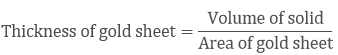⇒ 0.5 m3/1 Hectare

⇒ 0.5 m3/10000 m2

⇒ 100 m/20000

Therefore, Thickness of silver sheet = 1/200 cm

Question: 13

A metal cube of edge 12 cm is melted and formed into three smaller cubes. If the edges of the two smaller cubes are 6cm and 8cm, find the edge of the third smaller cube.

Solution:

Volume of the large cube = v1 + v2 + v3

Let the edge of the third cube be 'x' cm

123 = 63 + 83 + a3 [Volume of cube = side3]

1728 = 216 + 512 + x3

⇒ x3 = 1728 − 728 = 1000

⇒ x = 10 cm

Therefore, Side of third side = 10 cm

Question: 14

The dimensions of a cinema hall are 100 m, 50 m, 18 m. How many persons can sit in the hall, if each person requires 150 m3 of air?

Solution:

Given that

Volume of cinema hall = 100 * 50 * 18 m3

Volume of air required by each person = 150 m3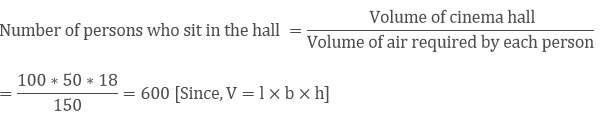Therefore, number of persons who can sit in the hall = 600 members.

Question: 15

Given that 1 cubic cm of marble weighs 0.25 kg, the weight of marble block 28 cm in width and 5 cm thick is 112 kg. Find the length of the block.

Solution:

Let the length of the marble block be 'l' cm

Volume of the marble block = l * b * h cm3

= l * 28 * 5 cm3

Therefore, weight of the marble square = 140l * 0.25 kg

As mentioned in the question, weight of the marble = 112 kgs

Therefore,

= 112 = 140l * 0.25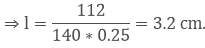Question: 16

A box with lid is made of 2 cm thick wood. Its external length, breadth and height are 25 cm, 18 cm and 15 cm respectively. How much cubic cm of a fluid can be placed in it? Also, find the volume of the wood used in it.

Solution:

Given,

The external dimensions of cuboid are as follows

Length (l) = 25 cm

Height (h) = 15 cm

External volume of the case with cover (cuboid) = l * b * h cm3

= 25 * 18 * 15 cm3

= 6750 cm3

Now, the internal dimensions of the cuboid is as follows

Length (l) = 25 - (2 * 2) = 21 cm

Breadth (b) = 18 - (2 * 2) = 14 cm

Height (h) = 15 - (2 * 2) = 11cm

Now, Internal volume of the case with cover (cuboid) = l * b * h cm3

= 21 * 14 * 11 cm3

= 3234 cm3

Therefore, Volume of the fluid that can be placed = 3234 cm3

Now, volume of the wood utilized = External volume – Internal volume

= 3516 cm3

Question: 17

The external dimensions of a closed wooden box are 48 cm, 36 cm, 30 cm. The box is made of 1.5 cm thick wood. How many bricks of size 6 cm × 3 cm × 0.75 cm can be put in this box?

Solution:

Given that,

The external dimensions of the wooden box are as follows:

Length (l) = 48 cm, Breadth (b) = 36 cm, Height (h) = 30 cm

Now, the internal dimensions of the wooden box are as follows:

Length (l) = 48 - (2 * 1.5) = 45 cm

Breadth (b) = 36 - (2 * 1.5) = 33 cm

Height (h) = 30 - (2 * 1.5) = 27 cm

Internal volume of the wooden box = l * b * h cm3

= 45 * 33 * 27 cm3

= 40095 cm3

Volume of the brick = 6*3*0.75 = 13.5 cm3

Therefore, Number of bricks = 40095/13.5 = 2970 bricks

Therefore, 2970 bricks can be kept inside the wooden box.

Question: 18

How many cubic centimeters of iron are there in an open box whose external dimensions are 36 cm, 25 cm and 16.5 cm, the iron being 1.5 cm thick throughout? If 1 cubic cm of iron weighs 15 gms. Find the weight of the empty box in kg.

Solution:

Given,

Outer dimensions of iron:

Length (l) = 36 cm

Height (h) = 16.5 cm

Inner dimensions of iron:

Length (l) = 36 - (2*1.5) = 33 cm

Breadth (b) = 25 - (2*1.5) = 22 cm

Height (h) = 16.5 - 1.5 = 15 cm

Volume of Iron = Outer volume – Inner volume

= (36 * 25 * 16.5) – (33 * 22 * 15)

= 3960 cm3

Weight of Iron = 3960 * 15 = 59400 grams = 59.4 kgs

Question: 19

A cube of 9 cm edge is immersed completely in a rectangular vessel containing water. If the dimensions of the base are 15 cm and 12 cm, find the rise in water level in the vessel.

Solution:

Volume of the cube = S3 = 93 = 729 cm3

Area of the base = l * b = 15 * 12 = 180 cm2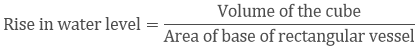= 729/180

= 4.05 cm

Question: 20

A rectangular container, whose base is a square of side 5cm, stands on a horizontal table, and holds water up to 1cm from the top. When a solid cube is placed in the water it is completely submerged, the water rises to the top and 2 cubic cm of water overflows. Calculate the volume of the cube and also the length of its edge.

Solution:

Let the length of each edge of the cube be ‘x’cm

Then, volume of the cube = Volume of water inside the tank + Volume of water that overflowed

x3 = (5 ∗ 5 ∗ 1) + 2

x3 = 27

x = 3 cm

Hence, volume of the cube = 27 cm3

And edge of the cube = 3 cm

Question: 21

A field is 200 m long and 150 m broad. There is a plot, 50 m long and 40 m broad, near the field. The plot is dug 7m deep and the earth taken out is spread evenly on the field. By how many meters is the level of the field raised? Give the answer to the second place of decimal.

Solution:

Volume of the earth dug out = 50 * 40 * 7 = 14000 m3

Let 'h' be the rise in the height of the field

Therefore, volume of the field (cuboidal) = Volume of the earth dug out

⇒ 200 ∗ 150 ∗ h = 14000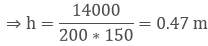Question: 22

A field is in the form of a rectangular length 18m and width 15m. A pit 7.5m long, 6m broad and 0.8m deep, is dug in a corner of the field and the earth taken out is spread over the remaining area of the field. Find out the extent to which the level of the field has been raised.

Solution:

Let 'h' metres be the rise in the level of field

Volume of earth taken out from the pit = 7.5 * 6 * 0.8 = 36 m3

Area of the field on which the earth taken out is to be spread = 18 * 15 - 7.5 * 6 = 225 m2

Now, Area of the field * h = Volume of the earth taken out from the pit

⇒ 225 ∗ h = 7.5 ∗ 6 ∗ 0.8

⇒ h = 36/225 = 0.16 m = 16 cm

Question: 23

A rectangular tank is 80 m long and 25 m broad. Water flows into it through a pipe whose cross-section is 25 cm2, at the rate of 16 km per hour. How much the level of the water rises in the tank in 45 minutes?

Solution:

Consider 'h' be the rise in water level.

Volume of water in rectangular tank = 8000 * 2500 * h cm2

Cross-sectional area of the pipe = 25 cm2

Water coming out of the pipe forms a cuboid of base area 25 cm2 and length equal to the distance travelled in 45 minutes with the speed 16 km/hour

i.e., length = Length = 16000 ∗ 100 ∗ 45/60 cm

Therefore, The Volume of water coming out pipe in 45 minutes = 25 * 16000 * 100 * (45/60)

Now, volume of water in the tank = Volume of water coming out of the pipe in 45 minutes

⇒ 8000 ∗ 2500 ∗ h = 16000 ∗ 100 ∗ 45/60 ∗ 25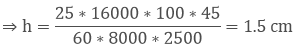Question: 24

Water in a rectangular reservoir having base 80 m by 60 m is 6.5m deep. In what time can the water be pumped by a pipe of which the cross-section is a square of side 20 cm if the water runs through the pipe at the rate of 15km/hr.

Solution:

Flow of water = 15 km/hr

= 15000 m/hr

Volume of water coming out of the pipe in one hour,

⇒ 20/100 ∗ 20/100 ∗ 15000 = 600 m3

Volume of the tank = 80 * 60 * 6.5

= 31200 m3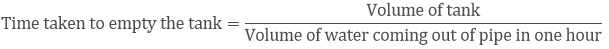= 31200/600 = 52 hours

Question: 25

A village having a population of 4000 requires 150 liters of water per head per day. It has a tank measuring 20 m × 15 m × 6 m. For how many days will the water of this tank last?

Solution:

Given that,

Length of the cuboidal tank (l) = 20 m

Breadth of the cuboidal tank (b) = 15 m

Height of the cuboidal tank (h) = 6 m

Capacity of the tank = l * b * h = 20 * 15 * 6

= 1800 m3

= 1800000 litres

Water consumed by the people of village in one day = 4000 *150 litres

= 600000 litres

Let water of this tank last for 'n' days

Therefore, water consumed by all people of village in n days = Capacity of the tank

= n*600000 = 1800000

= n = 1800000/600000 = 3

Thus, the water will last for 3 days in the tank.

Question: 26

A child playing with building blocks, which are of the shape of the cubes, has built a structure as shown in Fig. 18.12. If the edge of each cube is 3cm, find the volume of the structure built by the child.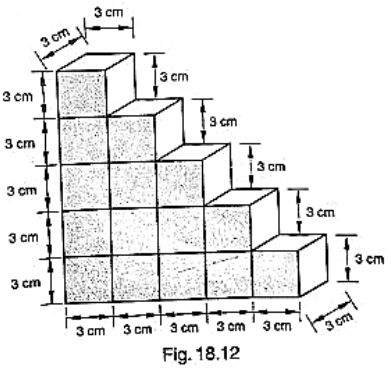Solution:

Volume of each cube = edge * edge * edge

= 3 * 3 * 3

= 27 cm3

Number of cubes in the structure = 15

Therefore, volume of the structure = 27*15

= 405 cm3

Question: 27

A godown measures 40 m × 25 m × 10 m. Find the maximum number of wooden crates each measuring 1.5 m × 1.25 m × 0.5 m that can be stored in the godown.

Solution:

Given,

Godown length (l1) = 40 m

Godown breadth (b1) = 25 m

Godown height (h1) = 10 m

Volume of the godown = l1 * b1 * h1 = 40 * 25 * 10

= 10000 m3

Wooden crate length (l2) = 1.5 m

Wooden crate breadth (b2) = 1.25 m

Wooden crate height (h2) = 0.5 m

Volume of the wooden crate = l2 * b2 * h2 = 1.5 * 1.25 * 0.5

= 0.9375 m3

The number of wooden crates stored in the godown is taken as 'n'

Volume of 'n' wooden crates = Volume of godown

= 0.9375 n = 10000

= n = 10000/0.9375 = 10666.66

Therefore, the number of wooden crates that can be stored in the godown is 10666.66.

Question: 28

A wall of length 10 m was to be built across an open ground. The height of the wall is 4m and thickness of the wall is 24 cm. If this wall is to be built up with bricks whose dimensions are 24 cm × 12 cm × 8 cm, how many bricks would be required?

Solution:

Given that,

The wall with all its bricks makes up space occupies by it, we need to find the volume of the wall, which is nothing but cuboid.

Here, length = 10 m = 1000 cm

Thickness = 24 cm

Height = 4 m = 400 cm

Therefore, volume of the wall = l * b * h

= 1000 * 24 * 400 cm3

Now, each brick is a cuboid with length = 24 cm

Height = 8 cm

So, volume of each brick = l * b * h = 24 * 12 * 8 = 2304 cm3

The number of bricks required is given by,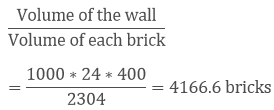So, the wall requires 4167 bricks.

```### Course Features

• 728 Video Lectures
• Revision Notes
• Previous Year Papers
• Mind Map
• Study Planner
• NCERT Solutions
• Discussion Forum
• Test paper with Video Solution